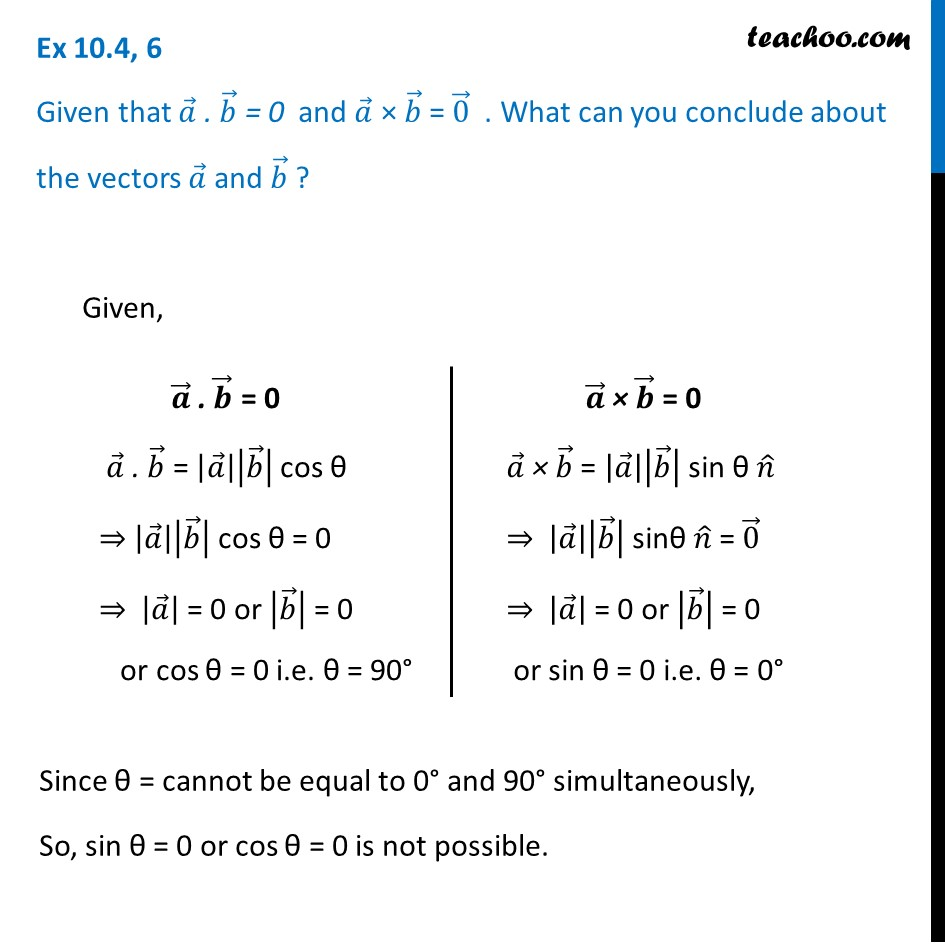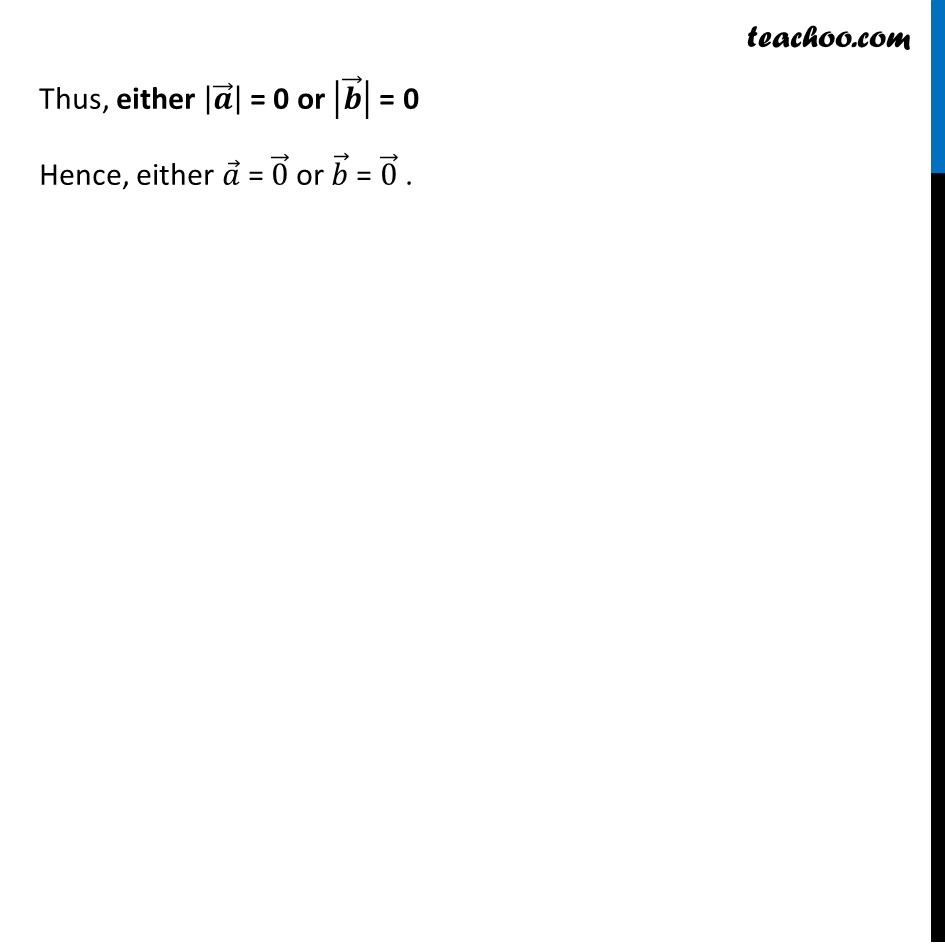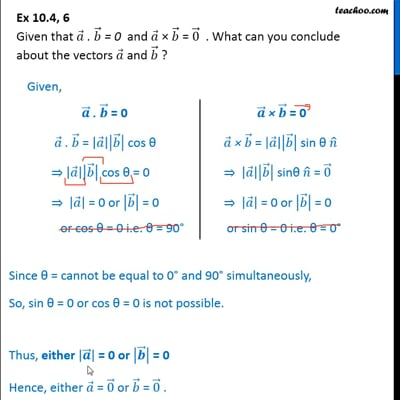Vector product - Solving

Chapter 10 Class 12 Vector Algebra
Concept wiseThis video is only available for Teachoo black users

Learn in your speed, with individual attention - Teachoo Maths 1-on-1 Class

### Transcript

Ex 10.4, 6 Given that 𝑎 ⃗ . 𝑏 ⃗ = 0 and 𝑎 ⃗ × 𝑏 ⃗ = 0 ⃗ . What can you conclude about the vectors 𝑎 ⃗ and 𝑏 ⃗ ? 𝒂 ⃗ . 𝒃 ⃗ = 0 𝑎 ⃗ . 𝑏 ⃗ = |𝑎 ⃗ ||𝑏 ⃗ | cos θ ⇒ |𝑎 ⃗ ||𝑏 ⃗ | cos θ = 0 ⇒ |𝑎 ⃗ | = 0 or |𝑏 ⃗ | = 0 or cos θ = 0 i.e. θ = 90° 𝒂 ⃗ × 𝒃 ⃗ = 0 𝑎 ⃗ × 𝑏 ⃗ = |𝑎 ⃗ ||𝑏 ⃗ | sin θ 𝑛 ̂ ⇒ |𝑎 ⃗ ||𝑏 ⃗ | sinθ 𝑛 ̂ = 0 ⃗ ⇒ |𝑎 ⃗ | = 0 or |𝑏 ⃗ | = 0 or sin θ = 0 i.e. θ = 0° Given, Since θ = cannot be equal to 0° and 90° simultaneously, So, sin θ = 0 or cos θ = 0 is not possible. Thus, either |𝒂 ⃗ | = 0 or |𝒃 ⃗ | = 0 Hence, either 𝑎 ⃗ = 0 ⃗ or 𝑏 ⃗ = 0 ⃗ .